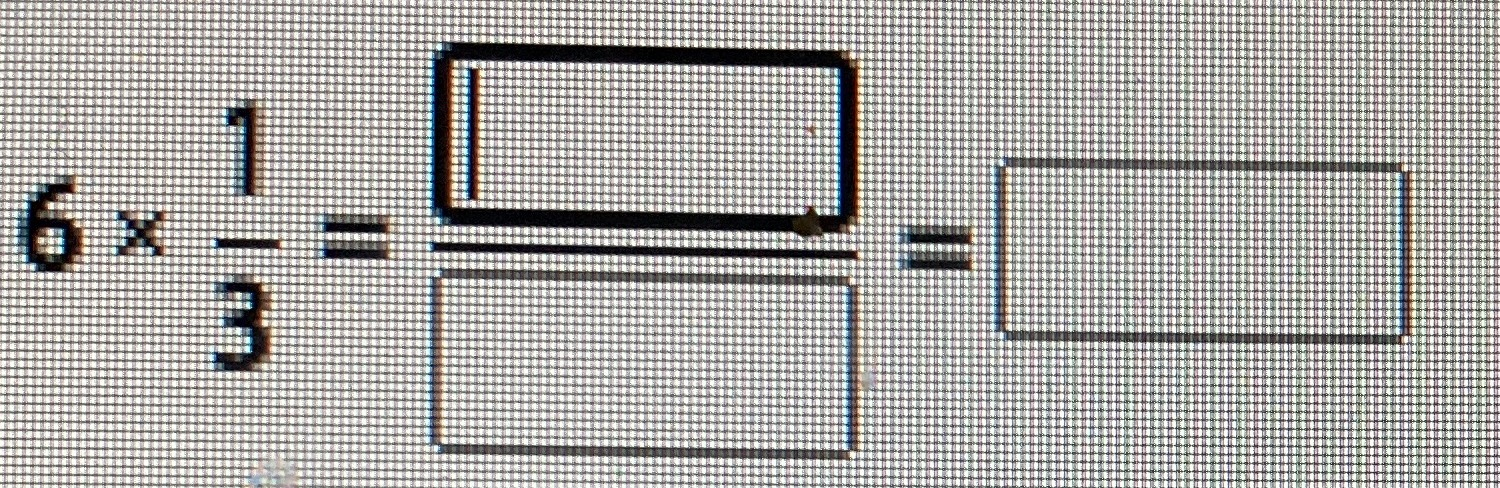Still have math questions?6$$\times$$$$\frac{1}{3}$$=$$\frac{6}{3}$$=2